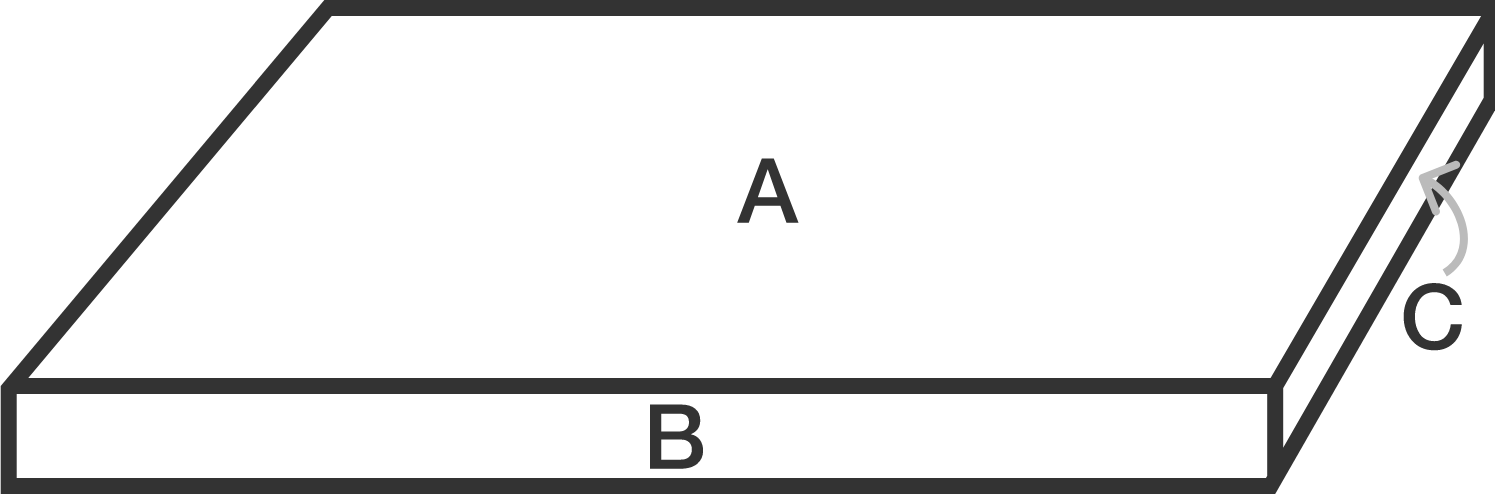# Curious Cuboid

Geometry Level 1The diagram above shows a rectangular prism. Given that the surface area of surface $A$, $B$, and $C$ are 1024, 81 and 49 respectively, find the volume of the cuboid.

×